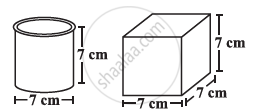Share

# Describe How the Two Figures at the Right Are Alike and How They Are Different. Which Box Has Larger Lateral Surface Area? - CBSE Class 8 - Mathematics

ConceptSurface Area and Volume of Cube, Cuboid and Cylinder Concept of Cube

#### Question

Describe how the two figures at the right are alike and how they are different. Which box has larger lateral surface area?#### Solution

Similarity between both the figures is that both have the same heights.

The difference between the two figures is that one is a cylinder and the other is a cube.

Lateral surface area of the cube = 4l2 = 4 (7 cm)2 = 196 cm2

Lateral surface area of the cylinder = 2πrh  = (2 xx 22/7 xx 7/2 xx 7) cm^2 = 154 cm2

Hence, the cube has larger lateral surface area.

Is there an error in this question or solution?

#### APPEARS IN

NCERT Solution for Mathematics Textbook for Class 8 (2018 to Current)
Chapter 11: Mensuration
Ex. 11.30 | Q: 6 | Page no. 186
Solution Describe How the Two Figures at the Right Are Alike and How They Are Different. Which Box Has Larger Lateral Surface Area? Concept: Surface Area and Volume of Cube, Cuboid and Cylinder - Concept of Cube.
S